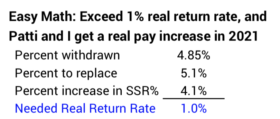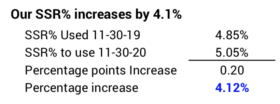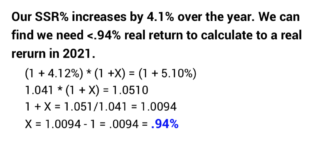abc

# What return on your nest egg do you need to get a pay increase in 2021?Posted on December 13, 2019Last week Patti and I calculated that we earned a small, real increase in our Safe Spending Amount (SSA) for 2020. I can now calculate that a 1% real return on our portfolio over this next year will result in another real increase in our SSA for 2021. Wow! A real pay increase with just 1% return on our portfolio. Sounds impossible. The purpose of this post is to show how I get to that 1% return rate. You’ll understand why it is so low. You will know how to quickly find the return on your portfolio that will give you a real pay increase for 2021.

== The quick math ==

The quick math that told me 1% real portfolio return would give us a real pay increase in our SSA for 2021 is the following: 1) I calculate the percentage return that earns back what we withdrew for spending for 2020: 1/(1-4.85%) – 1 = 5.1%. 2) I calculate how much of that we get from the increase in our applicable Safe Spending Rate (SSR%) from next year to this: (5.05% – 4.85%)/4.85% = 4.1%. 3) I subtract the two to get the real return needed: 5.1% – 4.1% = 1.0%. Read on for more detail.== Two factors work together ==

As I mentioned in this post, two factors work together to determine whether or not we nest eggers calculate to a greater real SSA – an increase beyond the normal one for inflation: 1) the real return rate on our portfolio and 2) the increase in our Safe Spending Rate (SSR%) applicable to our age.

To understand how these two factors work together, we have to think what’s happening in terms of real spending power: inflation will just confuse us. All dollars and return rates in this post are stated in terms of constant spending power.

The word “pay” that I use in this post is the amount Patti and I can spend after withholding all taxes – the after-tax amount from our gross SSA. I assume the total percentage taxes Patti and I pay does not change from this year to next. That means an increase in our total SSA also results in the same percentage increase in our pay.

If there is no change in our SSR%, Patti and I will calculate to an increase in our Safe Spending Amount (SSA) when we earn back more than the percentage we withdrew for our spending.

Example: Because of the high returns this year, Patti and I – like you – calculated to a new, higher SSA using the SSR% applicable to this year. In effect, we start on a new plan with a new beginning Investment Portfolio value, new SSR%, and multiplier (what we have relative to a base of \$1 million). (See Chapters 1 and 9, NEC.) For this post, let’s use assume the starting value of our Investment Portfolio is \$1 million and that we withdrew 4.85% SSR% = \$48,500. That left \$951,500 after the withdrawal. We earn back the \$48,500 – the 4.85% – with 5.1% real return on the \$951,500 portfolio.When our return is more than 5.1%, we’ll have more than \$1 million on November 30 next year. The same 4.85% SSR% will calculate to more than the current \$48,500 SSA – a real increase in our pay.

If we have 0% return on our portfolio in the year, Patti and I will calculate to the same \$48,500 SSA if our SSR% increases to 5.1%; this also works out to be a 5.1% increase in our SSR% percentage. Our SSR% increases in most – but not all – years, and it increases at a faster rate when we are older. This is similar to the way RMD works: RMD increases each year and it increases at a faster rate as we get older.

Example: we had \$951,500 after our withdrawal last week. If our return is 0% for the year ending November 30, 2020, we will have the same \$951,500. If our SSR% applicable to Patti’s age increases to 5.1% we would calculate to the same \$48,500 SSA.Conclusion: to calculate to a real increase in our Safe Spending Amount (SSA) next year, the Return Rate on our Investment Portfolio AND the percentage increase in our SSR% COMBINED must beat the percentage return that we would need to earn back the amount we withdrew from our portfolio. For Patti and me – withdrawing 4.85% – the combination of the two factors would need to beat 5.1%. If, for example, you withdrew 4.4% as your SSR% in 2020, you’d need to beat 4.6% in the upcoming year.== Two ways to find the real return you need ==

1. I can use our calculation sheet to find the real return over this next year that calculates to a pay increase for 2021. (You should have your own spreadsheet similar to the one I show in Appendix H of NEC; you can download a template from the Resources tab of this web site.)

I annotated our calculation sheet here so you can see how I found that we need 1% real return for a real pay increase in 2021. I had to enter numbers in two cells: 1) I set inflation to 0%. 2) Then I iterated to find the real return rate that will exceed our 2020 \$57,500 SSA: that was 4.85% of our portfolio value before that withdrawal. My iteration shows that .95% return first calculates to a real pay increase. Let’s just call that 1%.

(You can see an annotation on the sheet: if we earn the expected 6.4% real return on our portfolio for the 12 months ending November 30, 2020, we get a 5.4% real increase in our SSA.)

2. I can use straightforward math and not the spreadsheet. The math is simpler this year because we all start out, in effect, on a new plan: it’s a one-year calculation. As I mentioned in the same post above, the math to calculate to a real pay increase after a year – or more – of poor returns is conceptually the same, but it’s a bit more tedious to work out.

Patti and I withdrew 4.85% from our Investment Portfolio for our 2020 spending. The combination of real return rate and the percentage increase in our SSR% must yield more than 5.10%. We know our SSR% will increase next year from 4.85% to 5.05%. That’s a 4.10% increase; we get roughly 80% of the way to the 5.10% we are looking for:Now we have to calculate the real return rate gets us to the total of 5.10%.  We solve the unknown in the equation below and find that is .94%. This agrees with the number we found by using the spreadsheet: we need more than .94% to calculate to a real increase.== Use really quick math ==

You can quickly estimate the real return rate you need for a real increase in your SSA next year. You need three simple calculations to get close enough: 1) calculate the percent you would need to earn back if your SSR% did not change; 2) calculate the increase in your SSR% over the year; 3) subtract the two. You’ll be close enough.For Patti and me the simple steps would have been 1) 1/.9515 = 5.1%. 2) (5.05%-4.85%)/4.85% = 4.1%. 3) 5.1% – 4.1% = 1% real return needed for a real pay increase in 2021.

== Implications ==

Older retirees – generally those in their mid 70s – have a good chance of calculating to real pay increases even when portfolio returns are well below average. I did not think that would be the case. We’re taking out more when we are older because our SSR% is higher; I thought we would need a much greater return rate to justify increases in our SSA. I was not figuring in the effect of increasing SSR%: we older retirees get lots of help from the increasing SSR% applicable to our age. The graph 2-7 in NEC shows that SSR% starts to accelerate when our life expectancy is roughly 15 or so years. That’s going to be when we are in our early 70s.

Younger retirees in their early to mid 60s use a lower SSR% to calculate their SSA; they withdraw less for their SSA. The percentage they need to “get back” is less than for older retirees. But they aren’t getting much help from increases in their SSR%. They need greater real return rates to calculate to a real increase in SSA.

Conclusion: After we’ve calculated our Safe Spending Amount (SSA) for the upcoming year we can calculate the real return over the next 12 months that will result in a real increase in our SSA the following year. In most years real return needed is less than than the percentage you withdraw for your SSA. That’s because you are getting help from the fact that the Safe Spending Rate (SSR%) applicable to your age is increasing. The increase in SSR% for Patti and me over the next year is more than it has been in any other prior year. As a result, we only need a 1% real return on our portfolio for the 12 months ending November 30, 2020 to result is a real pay increase in 2021. This gives me a real sense of optimism.

WordPress Image Lightbox
WordPress Image Lightbox